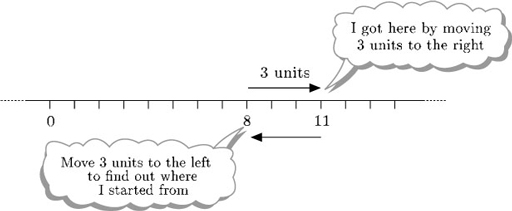The ECHR is essentially a charter of rights. Any charter of rights represents a consensus, a negotiated agreement between the drafters. Every state intending to adopt a charter will have its own vision and aims, and the drafters have to find a way of accommodating these visions and aims. This often results in the creation of provisions that are a compromise and are drafted in the widest possible terms. The ECHR is drafted in such a way. It is a vaguely worded aspirational charter inten
Author(s): The Open University

The Council of Europe was set up in 1949. It is an intergovernmental organisation (based in Strasbourg, France) set up to protect human rights, promote cultural diversity and to combat social problems such as intolerance. Its creation was seen as a way of achieving a European approach to the protection of certain individual rights. Although presented now as historical events, the horrors of what had taken place in the Second World War were then fresh in the minds of the governments and
Author(s): The Open University

Introduction to algebra
This free course is an introduction to algebra which builds on the idea of using letters to represent numbers. Section 1 looks at finding, simplifying and using formulas and includes useful tips on learning algebra. Section 2 introduces some of the terminology used in algebra and techniques such as evaluating and checking equivalence of expressions, identifying terms in an expression and collecting like terms. Section 3 looks at how to simplify terms and introduces index notation and multiplying
Author(s): Creator not set

Modelling and estimation
This free course is concerned with modelling and estimation and looks in particular at the binomial distribution. Section 1 starts by defining probability, introduces relevant notation and briefly discusses basic properties of probabilities. The section concludes by considering some of the general features of and ideas about modelling discrete random variables. Section 2 looks at one particular probability model for discrete data, the binomial distribution. Section 3 investigates how data can be
Author(s): Creator not set

Introduction to the calculus of variations
This free course concerns the calculus of variations. Section 1 introduces some key ingredients by solving a seemingly simple problem – finding the shortest distance between two points in a plane. The section also introduces the notions of a functional and of a stationary path. Section 2 describes basic problems that can be formulated in terms of functionals. Section 3 looks at partial and total derivatives. Section 4 contains a derivation of the Euler-Lagrange equation. In Section 5 the Euler
Author(s): Creator not set

Lastly consider division. Dividing 8 by 2 means ‘How many times does 2 go into 8?’ or ‘What must you multiply 2 by to get 8?’. The answer is 4.

So to find 8 ÷ 2, you need to ask ‘What do I have to multiply 2 by to get 8?’. The answer is 4, since 2 × 4 = 8.

So 8 ÷ 2 = 4.

Similarly, to find 8 ÷ 2 you need to ask ‘what do I have to mul
Author(s): The Open University

The rules for multiplying positive and negative numbers can be illustrated by the table below.

Multiplying a positive number by a positive number gives a positive answer.

Multiplying a negative number by a positive number gives a negative answer.

Multi
Author(s): The Open University

Now that you have rules for addition and subtraction of negative numbers, think about multiplication and division.

## Example 27

Describe each of the following in terms of the number line and the value of Thomas's piggy bank:

• (a) the mul
Author(s): The Open University

Now what about subtraction? You can think of subtraction as undoing addition: adding 3 to 8 gets you 11, and so subtracting 3 from the answer, 11, gets you back to 8. Therefore, in terms of the number line, subtracting 3 from 11 means starting at 11 and moving 3 units to the left.Author(s): The Open University

## Activity 52

Evaluate each of the following.

• [Image_Link]https://www.open.edu/openlearn/ocw/pluginfile.php/11742
Author(s):
The Open University

How about other fractions? What is 6 ÷? This means how many [Image_Link]https://www.open.edu/openlearn/ocw/pluginfile.php/117429/mod_ou
Author(s):
The Open University

## Activity 30

Carry out the following calculations, without your calculator.

• (a) 3 × (60 + 70).

• (b) (3 × 60) + 70.

• (c) (70 − 60) ÷ 5.

• Author(s): The Open University

## Activity 28

Look at the rules in the boxes above.

Write in your own words the rules for multiplying and dividing, adding and subtracting two numbers, giving an example of each.

Author(s): The Open University

In order to compare quantities, it is best to express them in the same units.

## Example 10

Three children have just measured their own heights in metric units. Isaac says ‘My height is 1098’, Jasmine says ‘My height is 112’ and Kim says ‘Mine is 1.1’. What units were
Author(s): The Open University

## Activity 15

Suggest appropriate units for each of the following:

• (a) the age of the kitten when it is weaned;

• (b) the distance between one train station and the
Author(s): The Open University

## Activity 9

The diagram below shows an oatmeal cake marked into 12 equal portions. I want to give my sister a third of the cake. Where could I cut the cake, and what would be left over?

Author(s): The Open University

In the example below of a straightforward subtraction, in every column the digit at the top of the column is bigger than the digit at the bottom. Click on each step in turn to see how to carry out the calculation.

Active content not displayed. This content requires JavaScript to be enabled.